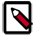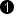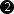## Operator DIV

The operator DIV divides the values at input link I1 by the values at input link I2. At output link O, the integer result of the division is provided. At output link R the remainder of the division is provided.

A division by zero is undefined at both outputs.

ResourcesA division requires many FPGA resources. Try to use a minimized parallelism when performing a division. For divisions by a power of two value (2^n) use the shift operator ShiftRight instead.

### I/O Properties

Property Value
Operator Type O
Input Links I1, dividend / numerator input
I2, divisor / denominator input
Output Links O, integer quotient output
R, remainder output

Bit Width [1, 64] [1, 64]autoas I2
Arithmetic {unsigned, signed} as I as I as I
Parallelism any as I as I as I
Kernel Columns any as I as I as I
Kernel Rows any as I as I as I
Img Protocol {VALT_IMAGE2D, VALT_LINE1D, VALT_PIXEL0D} as I as I as I
Color Format VAF_GRAY as I as I as I
Color Flavor FL_NONE as I as I as I
Max. Img Width any as I as I as I
Max. Img Height any as I as I as I
 The bit width of the input I2 (divisor) has to be less equal than the bitwidth of the input at link I1 (dividend). For unsigned inputs the bit width at output O is equal to the bitwidth at I1. For signed inputs, the bit width is equal to the bit width at I1 + 1.

None

### Examples of Use

The use of operator DIV is shown in the following examples:

• 'Laser Triangulation'

Examples - A high speed and robust laser line detection algorithm. The algorithm determines center of gravity coordinates to obtain sub-pixel resolution results.

• 'Blob2D ROI Selection'

Examples - The blob analysis operator is applied to an input camera image. The applet shows the usage of the blob data in the applet. In this case, the object with the maximum are is localized and the coordinates are used to cut out the object from the original image.

• 'Moments in Image Processing'

Example - Calculates image moments orientation and eccentricity

• 'Shear of an Image'

Example - Line Shear example with linear interpolation.